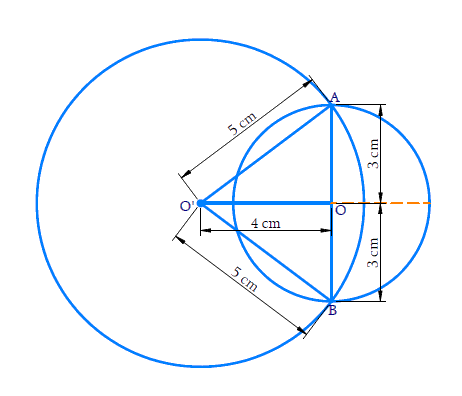In the verge of coronavirus pandemic, we are providing FREE access to our entire Online Curriculum to ensure Learning Doesn't STOP!

# Ex.10.4 Q1 Circles Solution - NCERT Maths Class 9

Go back to  'Ex.10.4'

## Question

Two circles of radii $$5 \rm{cm}$$ and $$3 \rm{cm}$$ intersect at two points and the distance between their centers is $$4 \rm{cm}.$$ Find the length of the common chord.

Video Solution
Circles
Ex 10.4 | Question 1

## Text Solution

What is known?

Radii of two circles and distance between the centers of the circles.

What is unknown?

Length of common chord.

Reasoning:

Perpendicular bisector of the common chord passes through the centers of both the circles.

Steps:Given that the circles intersect at $$2$$ points, so we can draw the above figure.

Let $$AB$$ be the common chord. Let $$O$$ and $$O^\prime$$ be the centres of the circles respectively.

\begin{align} &{O}^{\prime} {A}=5 {\rm{cm}}, {OA}=3 {\rm{cm}},\\ & {OO}^{\prime}=4 {\rm{cm}}\end{align}

Since the radius of the bigger circle is more than the distance between the $$2$$ centres, we can say that the centre of smaller circle lies inside the bigger circle itself.

$${OO}’$$ is the perpendicular bisector of $${AB.}$$

\begin{align} {\rm{So,}} \;{OA}&={OB}=3 \,\rm{cm}\\ {AB}&=3+3=6\, \rm{cm} \end{align}

Length of the common chord is $$6 \,\rm{cm.}$$

It is also evident that common chord is the diameter of the smaller circle.

Video Solution
Circles
Ex 10.4 | Question 1

Learn from the best math teachers and top your exams

• Live one on one classroom and doubt clearing
• Practice worksheets in and after class for conceptual clarity
• Personalized curriculum to keep up with school